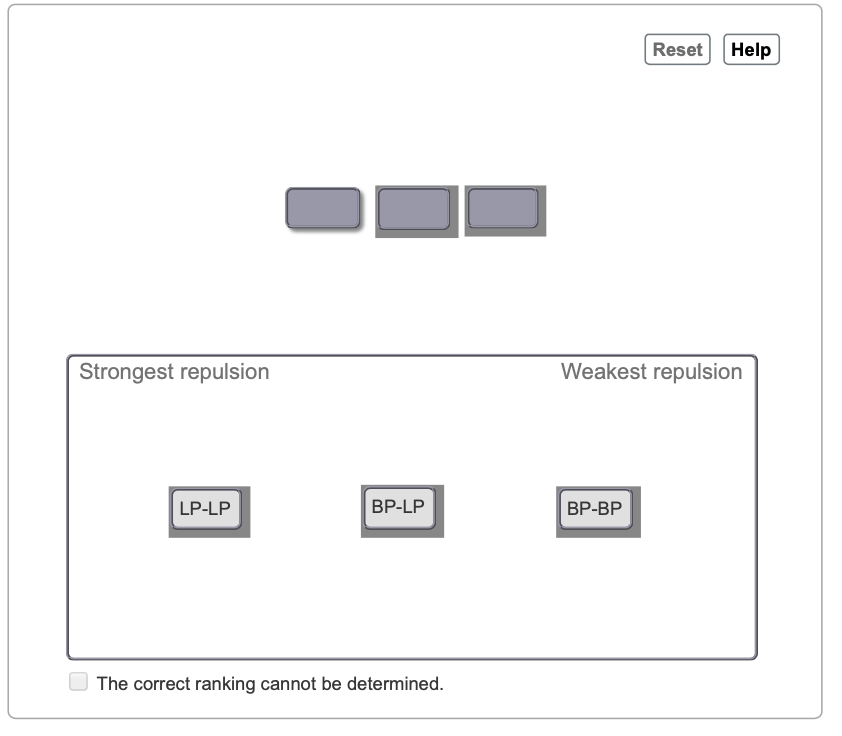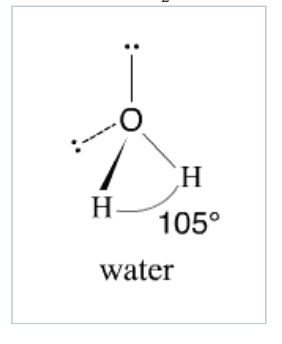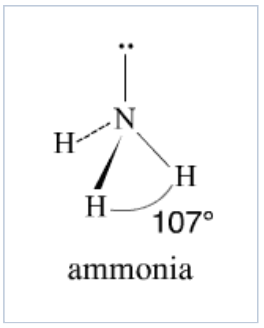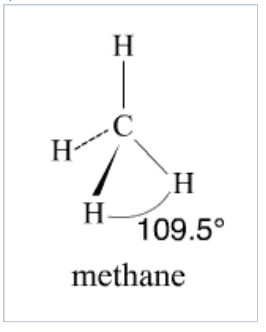Problem: Part A. Based on the bond angles in CH4, NH3, and H2O, rank the magnitude of these repulsions. Rank from strongest to weakest repulsion. To rank items as equivalent, overlap them.According to valence-shell electron-pair repulsion (VSEPR) theory, electron pairs around a central atom repel each other. This accounts for the geometries, or shapes, of molecules. For example, CH4 is tetrahedral, with all four outer hydrogen atoms spaced 109.5° from each other, as shown here. This geometry results from the fact that the bonding electron pairs repel each other equally and therefore remain as far apart as possible.NH3, with three outer atoms and one lone pair, also has a tetrahedral electronic geometry. However, the bond angles in NH3 are only 107°. This indicates that the magnitude of repulsion between a lone pair and a bonded pair of electrons must be different from the magnitude of repulsion between two bonded pairs of electrons.H2O, with two outer atoms and two lone pairs, also has a tetrahedral electronic geometry. However, the bond angles in H2O are only 105°. This indicates that the magnitude of repulsion between two lone pairs must be different from the magnitude of repulsion between a lone pair and a bonded pair of electrons.If BP stands for a bonded pair of electrons and LP stands for a lone pair of electrons, then BP-BP represents the repulsion between two bonded pairs, LP-LP represents the repulsion between two lone pairs, and BP-LP represents the repulsion between a bonded pair and a lone pair of electrons.

FREE Expert Solution
99% (394 ratings)Problem Details

Part A. Based on the bond angles in CH4, NH3, and H2O, rank the magnitude of these repulsions.Rank from strongest to weakest repulsion. To rank items as equivalent, overlap them.

According to valence-shell electron-pair repulsion (VSEPR) theory, electron pairs around a central atom repel each other. This accounts for the geometries, or shapes, of molecules. For example, CH4 is tetrahedral, with all four outer hydrogen atoms spaced 109.5° from each other, as shown here. This geometry results from the fact that the bonding electron pairs repel each other equally and therefore remain as far apart as possible.

NH3, with three outer atoms and one lone pair, also has a tetrahedral electronic geometry. However, the bond angles in NH3 are only 107°. This indicates that the magnitude of repulsion between a lone pair and a bonded pair of electrons must be different from the magnitude of repulsion between two bonded pairs of electrons.

H2O, with two outer atoms and two lone pairs, also has a tetrahedral electronic geometry. However, the bond angles in H2O are only 105°. This indicates that the magnitude of repulsion between two lone pairs must be different from the magnitude of repulsion between a lone pair and a bonded pair of electrons.If BP stands for a bonded pair of electrons and LP stands for a lone pair of electrons, then BP-BP represents the repulsion between two bonded pairs, LP-LP represents the repulsion between two lone pairs, and BP-LP represents the repulsion between a bonded pair and a lone pair of electrons.